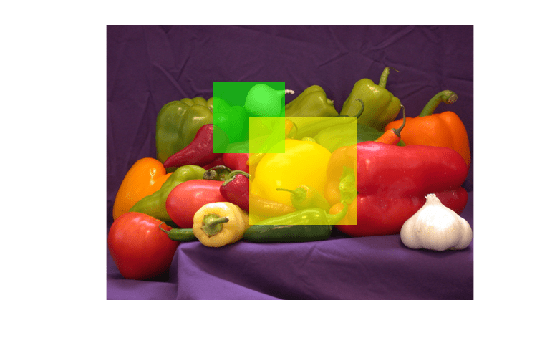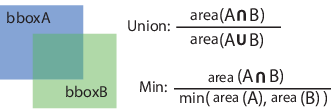Main Content

# bboxOverlapRatio

Compute bounding box overlap ratio

## Syntax

``overlapRatio = bboxOverlapRatio(bboxA,bboxB)``
``overlapRatio = bboxOverlapRatio(bboxA,bboxB,ratioType)``

## Description

example

````overlapRatio = bboxOverlapRatio(bboxA,bboxB)` returns the overlap ratio between each pair of bounding boxes `bboxA` and `bboxB`. The function returns the `overlapRatio` value between `0` and `1`, where `1` implies a perfect overlap.```
````overlapRatio = bboxOverlapRatio(bboxA,bboxB,ratioType)` additionally lets you specify the method to use for computing the ratio. You must set the `ratioType` to either `'Union'` or `'Min'`.```

## Examples

collapse all

Define two bounding boxes in the format [x y width height].

```bboxA = [150,80,100,100]; bboxB = bboxA + 50;```

Display the bounding boxes on an image.

```I = imread('peppers.png'); RGB = insertShape(I,'FilledRectangle',bboxA,'Color','green'); RGB = insertShape(RGB,'FilledRectangle',bboxB,'Color','yellow'); imshow(RGB)```Compute the overlap ratio between the two bounding boxes.

`overlapRatio = bboxOverlapRatio(bboxA,bboxB)`
```overlapRatio = 0.0833 ```

Randomly generate two sets of bounding boxes.

```bboxA = 10*rand(5,4); bboxB = 10*rand(10,4);```

Ensure that the width and height of the boxes are positive.

```bboxA(:,3:4) = bboxA(:,3:4) + 10; bboxB(:,3:4) = bboxB(:,3:4) + 10;```

Compute the overlap ratio between each pair.

`overlapRatio = bboxOverlapRatio(bboxA,bboxB)`
```overlapRatio = 5×10 0.2431 0.2329 0.3418 0.5117 0.7972 0.1567 0.1789 0.4339 0.0906 0.5766 0.3420 0.1655 0.7375 0.5188 0.2786 0.3050 0.2969 0.4350 0.2477 0.2530 0.4844 0.3290 0.3448 0.1500 0.1854 0.4976 0.5629 0.4430 0.5027 0.2685 0.3681 0.0825 0.3499 0.0840 0.0658 0.5921 0.6498 0.1930 0.7433 0.0676 0.3752 0.1114 0.3114 0.0696 0.0654 0.5408 0.6234 0.2046 0.7557 0.0717 ```

## Input Arguments

collapse all

Bounding boxes, specified as an M-by-4 or M-by-5 nonsparse numeric matrix. M is the number of bounding boxes. Each row of the matrix defines a bounding box as either an axis-aligned rectangle or a rotated rectangle. This table describes the format for each bounding box.

Bounding BoxRowDescription
Axis-aligned rectangle[xmin, ymin, width, height]This type of bounding box is defined in pixel coordinates as an M-by-4 matrix representing M bounding boxes
Rotated rectangle[xcenter, ycenter, width, height, yaw]This type of bounding box is defined in spatial coordinates as an M-by-5 matrix representing M bounding boxes. The xcenter and ycenter coordinates represent the center of the bounding box. The width and height elements represent the length of the box along the x and y axes, respectively. The yaw represents the rotation angle in degrees. The amount of rotation about the center of the bounding box is measured in the clockwise direction.

Data Types: `single` | `double` | `int8` | `int16` | `int32` | `uint8` | `uint16` | `uint32`

Bounding boxes, specified as an M-by-4 or M-by-5 nonsparse numeric matrix. M is the number of bounding boxes. Each row, M, of the matrix defines a bounding box as either an axis-aligned rectangle or a rotated rectangle. This table describes the format for each bounding box.

Bounding BoxRowDescription
Axis-aligned rectangle[xmin, ymin, width, height]This type of bounding box is defined in pixel coordinates as an M-by-4 matrix representing M bounding boxes
Rotated rectangle[xcenter, ycenter, width, height, yaw]This type of bounding box is defined in spatial coordinates as an M-by-5 matrix representing M bounding boxes. The xcenter and ycenter coordinates represent the center of the bounding box. The width and height elements represent the length of the box along the x and y axes, respectively. The yaw represents the rotation angle in degrees. The amount of rotation about the center of the bounding box is measured in the clockwise direction.

Data Types: `single` | `double` | `int8` | `int16` | `int32` | `uint8` | `uint16` | `uint32`

Ratio type, specified as the character vector `'Union'` or `'Min'`.

• Set the ratio type to `'Union'` to compute the ratio as the area of intersection between `bboxA` and `bboxB`, divided by the area of the union of the two.

• Set the ratio type to `'Min'` to compute the ratio as the area of intersection between `bboxA` and `bboxB`, divided by the minimum area of the two bounding boxes.Data Types: `char`

## Output Arguments

collapse all

Overlap ratio between two bounding boxes, returned as an M-by-N matrix. Each (I, J) element in the output matrix corresponds to the overlap ratio between row I in `bboxA` and row J in `bboxB`. The function returns `overlapRatio` in the between 0 and 1, where `1` implies a perfect overlap. If either `bboxA` or `bboxB` is double, then the function returns `overlapRatio` as `double`. Otherwise, the function returns it as `single`.

The function computes the overlap ratio based on the ratio type. You can set `ratioType` to `'Union'` or `'Min'`:Data Types: `single` | `double`

## See Also

### Topics

Introduced in R2014b

Download ebook